Electron. J. Diff. Equ., Vol. 2012 (2012), No. 101, pp. 1-14.

### Positivity and negativity of solutions toweighted systems involving the Laplace operator on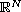Benedicte Alziary, Jacqueline Fleckinger, Marie-Helene Lecureux, Na Wei

Abstract:
We consider the sign of the solutions of asystem defined on the whole space,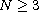and a weight functionwith a positive part decreasing fast enough,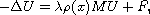where F is a vector of functions, M is amatrix with constant coefficients, not necessarily cooperative, and the weight functionis allowed to change sign. We prove that the solutions of thesystem exist and then we prove the local fundamental positivity and local fundamental negativity of the solutions when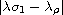is small enough, where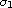is the largest eigenvalue of the constant matrix M and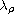is the "principal" eigenvalue of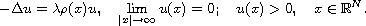Submitted February 29, 2012. Published June 15, 2012.
Math Subject Classifications: 35B50, 35J05, 35J47.
Key Words: Elliptic PDE; maximum principle; fundamental positivity; fundamental negativity; indefinite weight, weighted systems.

Show me the PDF file (302 KB), TEX file, and other files for this article.Bénédicte Alziary Institut de Mathématique -UMR CNRS 5219- et Ceremath-UT1 Université de Toulouse 31042 Toulouse Cedex, France email: alziary@univ-tlse1.fr Jacqueline Fleckinger Institut de Mathématique -UMR CNRS 5219- et Ceremath-UT1 Université de Toulouse 31042 Toulouse Cedex, France email: jfleckinger@gmail.com Marie-Hélène Lecureux Institut de Mathématique -UMR CNRS 5219- et Ceremath-UT1 Université de Toulouse 31042 Toulouse Cedex, France email: mhlecureux@gmail.com Na Wei Dept. Appl. Math., Northwestern Polytechnical Univ. 710072 Xi'an, China School of Stat. & Math., Zhongnan Univ. Eco. & Law 430073, Wuhan, China email: nawei8382@gmail.com